Šajā tīmekļa vietnē tiek izmantotas sīkdatnes. Turpinot lietot šo vietni, jūs piekrītat sīkdatņu izmantošanai. Uzzināt vairāk.
LEGAL ACTS OF THE REPUBLIC OF LATVIA
home

The translation of this document is outdated.
Translation validity: 01.01.2018.–12.01.2022.
Amendments not included: 11.01.2022.
 Republic of Latvia Cabinet Regulation No. 765 Adopted 19 December 2017

Procedures by which the Manager of Funds of the State Funded Pension Scheme shall Calculate the Payment for the Management of an Investment Plan and Procedures for the Accounting and Deduction of the Abovementioned Payment

Issued pursuant to
Section 11, Paragraph 5.1, Clause 2
and Section 11, Paragraph 5.2
of the Law on State Funded Pensions

I. General Provisions

1. This Regulation prescribes:

1.1. the procedures by which the manager of funds of the State funded pension scheme (hereinafter - the manager) shall calculate the fixed and variable part of the payment for the management of an investment plan (hereinafter - the payment);

1.2. the procedures for the accounting and deduction of the fixed and variable part of the payment.

2. Terms used in this Regulation:

2.1. day of calculation - the day for which the value of an investment plan and the value of the part of an investment plan have been determined;

2.2. part value (DV) - the value of an investment plan part on the day of calculation after deduction of the fixed and variable part of the payment;

2.3. incomplete value of a part (DVN) - the value of a part of an investment plan on the day of calculation prior to deduction of the variable part of the payment;

2.4. reference index - an index which is used for comparing the DVN increase in (DVNP) and for calculating the variable part of the payment. The reference index is based upon the combination of indices of debt securities (hereinafter - the bonds) and equity securities (hereinafter - the shares);

2.5. start date of the period - the date which is used for calculating increase in the incomplete value of a part(DVNP) and the reference index increase (AIP). The start date of the period shall be:

2.5.1. the previous day of calculation if the calculation is made for one day of calculation;

2.5.2. the last day of calculation of the preceding year if the calculation is made for the period from the beginning of the year;

2.5.3. the day of calculation of the same date eight years ago or if there is no day of calculation for the respective date, the first available day of calculation prior to the day of calculation of the same date eight years ago, if the calculation has been made for a period of eight years.

3. The DVN increase (DVNP) earned or lost by an investment plan prior to deduction of the variable part of the payment on the day of calculation shall be calculated, using the following formula:

 DVNPt = DVNt -1 , where DV0

DVNPt - DVNP on the day of calculation in accordance with the time periods referred to in Sub-paragraph 2.5 of this Regulation;

DVNt - DVN of the day of calculation;

DV0 - DV of the start date of the period.

4. The reference index increase (AIP) shall be calculated, using the following formula:

 AIPt = (1 - KAP) × ( LBEATREUt -1) + KAP × ( SXXRt -1) , where LBEATREU0 SXXR0

AIPt - AIP of the period on the day of calculation in accordance with the time periods referred to in Sub-paragraph 2.5 of this Regulation;

LBEATREUt - the index of the bonds of the day of calculation Bloomberg Barclays Euro Aggregate Total Return Euro Unhedged (LBEATREU Index, FIGIBBG002SG6D04);

LBEATREU0 - the index of the bonds of the start date of the period Bloomberg Barclays Euro Aggregate Total Return Euro Unhedged in accordance with the time periods referred to in Sub-paragraph 2.5 of this Regulation;

SXXRt - the index of the shares of the day of calculation STOXX Europe 600 Net Return (SXXR Index; FIGI BBG000P5N0N9; ISIN EU0009658210);

SXXR0 - the index of the shares of the start date of the period STOXX Europe 600 Net Return in accordance with the time periods referred to in Sub-paragraph 2.5 of this Regulation;

KAP - the share index part which is specified according to the maximum share of investments provided for in the investment plan prospectus for capital securities, alternative investment funds or such investment funds that can make investments in capital securities or other financial instruments of equivalent risk:

KAP = 0.54, if the share varies from 50 % (non-inclusive) up to 75 % (inclusive);

KAP = 0.36, if the share varies from 25 % (non-inclusive) up to 50 % (inclusive);

KAP = 0.18, if the share varies from 0 % (non-inclusive) up to 25 % (inclusive);

KAP = 0.0, if such investments are not provided for in the plan prospectus.

5. If the index of bonds or shares is not available on the day of calculation, the last known value of the respective index prior to this day shall be used.

6. If any of the indices used in calculations is terminated, the manager shall replace the respective index in calculations as follows:

6.1. the index Bloomberg Barclays Euro Aggregate Total Return Euro Unhedged is replaced with the index Euro Broad Investment-Grade Bond Index (EuroBIG) (SBEB Index, FIGI BBG002SBH4Y6);

6.2. the index Euro Broad Investment-Grade Bond Index (EuroBIG) is replaced with the index iBoxx Euro Eurozone Sovereign 5-7 Total Return Index (QW1M Index, FIGI BBG000RFTW22);

6.3. the index STOXX Europe 600 Net Return is replaced with the index S&P Europe 350 Net Total Return Index (SPTR350N Index, FIGI BBG002SSHQ07);

6.4. the index S&P Europe 350 Net Total Return Index is replaced with the index EURO STOXX 50 Net Return EUR (SX5T Index, FIGI BBG000P5MXR4).

7. Excess profit - the difference between DVNP and AIP - shall be calculated, using the following formula:

VPt = DVNPt - AIPt , where

VPt - excess profit of the period on the day of calculation;

DVNPt - DVNP on the day of calculation;

AIPt - AIP of the period on the day of calculation.

8. The result of calculations referred to in Paragraphs 3, 4, and 7 of this Regulation shall be rounded up by the manager with the accuracy of seven digits after the comma.

II. Calculation, Accounting and Deduction of the Fixed Part of the Payment

9. The manager shall ensure accounting of the fixed part of the payment specified in the Law on State Funded Pensions in the financial reports of an investment plan for each calendar day and shall calculate it, using the following formula:

 MPDt = PAVt-1 × MP × N , where D

MPDt - the amount of the fixed part of the payment on the day of calculation;

PAVt-1 - net asset value of an investment plan on the previous day of calculation;

MP - the fixed part of the payment in percentage that has been specified in accordance with Section 11, Paragraph 5.1 of the Law on State Funded Pensions;

D - the number of calendar days in the reporting year;

N - the number of calendar days counting from the previous day of calculation.

10. The fixed part of the payment shall be accrued over the time period of one month, obtaining the total amount of the fixed part of the payment for the current month, and it shall be calculated by summing up the daily fixed part of the payment calculated for each day of calculation from the beginning of the current month, using the following formula:

 MPDK = K MPDt , where ∑ t = 1

MPDK - the amount of the fixed part of the payment for the current month;

MPDt - the amount of the fixed part of the payment for each day of calculation;

K - the number of day of calculations in the current month.

11. The manager shall deduct the fixed part of the payment for the current month once every month.

III. Calculation, Accounting and Deduction of the Variable Part of the Payment

12. The calculation of the variable part of the payment shall cover the maximum amount of the variable part of the payment accrued for the intermediate result, the variable part of the payment calculated for the intermediate result, and the variable part of the payment accounted in financial reports of the investment plan from the beginning of the year until the day of calculation (inclusive).

13. The manager shall proceed as follows with the maximum amount of the variable part of the payment accrued for the intermediate result (MMD):

13.1. calculate in euros for each calendar year, using the following formula:

 MMDt = PAVt-1 × (MK - MP) × N , where D

MMDt - the maximum amount of the variable part of the payment on the day of calculation;

PAVt-1 - net asset value of an investment plan on the previous day of calculation;

MK - the maximum annual payment in percentage which has been specified in accordance with Section 11, Paragraph 5.3 of the Law on State Funded Pensions;

MP - the fixed part of the payment in percentage which has been specified in accordance with Section 11, Paragraph 5.1 of the Law on State Funded Pensions;

D - the number of calendar days in the reporting year;

N - the number of calendar days counting from the previous day of calculation;

13.2. accrue during the time period of one year, obtaining the total MMD for the current year to be calculated by summing up the MMD calculated for each day of calculation from the beginning of the current year, using the following formula:

 MMDK = K MMDt , where ∑ t = 1

MMDK - the maximum amount of the variable part of the payment for the current year;

MMDt - the maximum amount of the variable part of the payment for each day of calculation;

K - the number of days of calculation in the current year;

13.3. start the accrual anew each year. The MMD prior to the calculation of the MMD on the first day of calculation of the current year shall be equal to 0.

14. The manager shall proceed as follows with the variable part of the payment calculated for the intermediate result (AMMD):

14.1. calculate for each day of calculation;

14.2. if excess profit on this day for the period of the past eight years exceeds zero, calculate for the day of calculation, using the following formula:

AMMDt = PAVt-1 × 20 % × min (VP1dt;VP8gt) , where

AMMDt - the amount of the calculated variable part of the payment for each day of calculation;

PAVt-1 - net asset value of an investment plan on the previous day of calculation;

VP1dt - excess profit of the day of calculation on the day of calculation;

VP8gt - excess profit of eight years on the day of calculation;

14.3. accrue during tie time period of one year, obtaining the total amount of the AMMD for the current year to be calculated by summing up the amount of the AMMD calculated for each day of calculation from the beginning of the current year, using the following formula:

 AMMDK = K AMMDt , where ∑ t = 1

AMMDK - the accrued amount of the calculated variable part of the payment for the current year;

AMMDt - the amount of the calculated variable part of the payment for each day of calculation;

K - the number of days of calculation in the current year;

14.4. if excess profit on this day for the period of the past eight years is equal to zero or less, calculate for the day of calculation, using the following formula:

AMMDt = min(0; 0 - AMMDK,t-1), where

AMMDt - the amount of the calculated variable part of the payment for each day of calculation;

AMMDK,t-1 - the accrued amount of the calculated variable part of the payment for the current year on the previous day of calculation;

14.5. start the accrual anew each year. The amount of the AMMD prior to the calculation of the AMMD on the first day of calculation of the current year shall be equal to 0.

15. The manager shall proceed as follows with the variable part of the payment accounted in financial reports of the investment plan (UMMD):

15.1. calculate for the current year on the day of calculation, using the following formula:

UMMDK = max(0; min(MMDK; AMMDK)) , where

UMMDK - the amount of the variable part of the payment accounted in financial reports of the investment plan for the current year;

MMDK - the maximum amount of the variable part of the payment for the current year;

AMMDK - the accrued amount of the calculated variable part of the payment for the current year;

15.2. calculate for the day of calculation on the first day of calculation of the year, using the following formula:

UMMDt = UMMDK,t , where

UMMDt - the amount of the variable part of the payment accounted in financial reports of the investment plan for the day of calculation;

UMMDK,t - the amount of the variable part of the payment accounted in financial reports of the investment plan for the current year on the day of calculation;

15.3. calculate for other days of calculation, using the following formula:

UMMDt = UMMDK,t - UMMDK,t-1 , where

UMMDt - the amount of the variable part of the payment accounted in financial reports of the investment plan for the day of calculation;

UMMDK,t - the amount of the variable part of the payment accounted in financial reports of the investment plan for the current year on the day of calculation;

UMMDK,t-1 - the amount of the variable part of the payment accounted in financial reports of the investment plan for the current year on the previous day of calculation;

15.4. if on the last day of calculation of the year the DV is equal to or less than the DV of the last day of calculation of the year in the time period of the past seven years prior to the current year, but the amount of the UMMD for the current year is positive, calculate, using the following formula:

UMMDt = 0 - UMMDK,t-1 , where

UMMDt - the amount of the variable part of the payment accounted in financial reports of the investment plan for the day of calculation;

UMMDK,t-1 - the amount of the variable part of the payment accounted in financial reports of the investment plan for the current year on the previous day of calculation.

16. The manager shall deduct the total amount of the variable part of the payment for the current year once every year.

IV. Closing Provisions

17. In 2018, 2019, 2020, 2021, 2022, 2023, 2024, and 2025, 31 December 2017 shall be considered as the start date of the time period for calculations made for the time period of eight years.

18. 31 December 2017 shall be determined as the last day of calculation of 2017 for all investment plans.

19. The accounting of the period of the preceding seven years referred to in Sub-paragraph 15.4 of this Regulation shall be commenced from 31 December 2017 (inclusive).

20. In respect of investment plans launched after the date of coming into force of this Regulation, the first day of calculation of these plans shall be considered as the start date of the period in calculations made about the time period of the initial eight years of this investment plan and also in calculations made during the first calendar year of these investment plans for the time period from the beginning of the year.

21. Cabinet Regulation No. 615 of 14 October 2014, Procedures by which the Manager of Funds of the State Funded Pension Scheme shall Calculate the Payment for the Management of an Investment Plan and Procedures for the Accounting and Deduction of the Abovementioned Payment (Latvijas Vēstnesis, 2014, No. 205), is repealed.

22. This Regulation shall come into force on 1 January 2018.

Prime Minister Māris Kučinskis

Acting for the Minister for Welfare,
Minister for Health Anda Čakša

Translation © 2018 Valsts valodas centrs (State Language Centre)

Document information
Status:
in force
Issuer: Cabinet of Ministers Type: regulation Document number: 765Adoption: 19.12.2017.Entry into force: 01.01.2018.Publication: Latvijas Vēstnesis, 254, 21.12.2017. OP number: 2017/254.14
Related documents
• Amendments
• Changes legal status of
• Issued pursuant to
• Annotation / draft legal act
• Other related documents
• Ar grozījumu sarakstu
• Ar manām piezīmēm
• Ar lielāka izmēra fontu
• Drukāt tiesību aktu
• Draugiem.lv

Latvijas Vestnesis, the official publisher
ensures legislative acts systematization
function on this site.
All Likumi.lv content is intended for information purposes.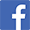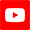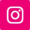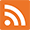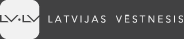"Everyone has the right to know about his or her rights." Article 90 of the Constitution of the Republic of Latvia © Official publisher "Latvijas Vēstnesis" ISO 9001:2015 (quality management system) ISO 27001:2013 (information security)